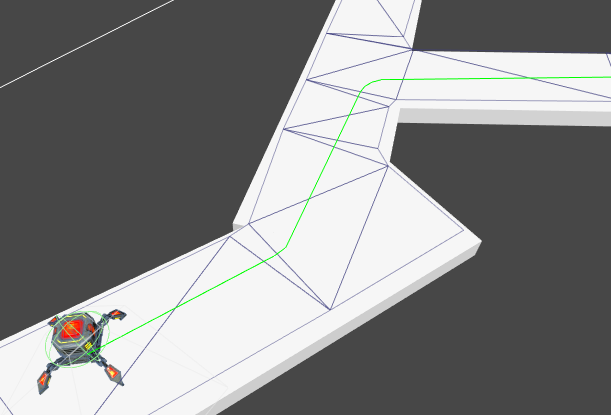A* Pathfinding Project  4.1.12 The A* Pathfinding Project for Unity 3D

Radius path modifier for offsetting paths. More...

## Detailed Description

Radius path modifier for offsetting paths.

The radius modifier will offset the path to create the effect of adjusting it to the characters radius. It gives good results on navmeshes which have not been offset with the character radius during scan. Especially useful when characters with different radiuses are used on the same navmesh. It is also useful when using rvo local avoidance with the RVONavmesh since the RVONavmesh assumes the navmesh has not been offset with the character radius.

This modifier assumes all paths are in the XZ plane (i.e Y axis is up).

It is recommended to use the Funnel Modifier on the path as well.RVONavmesh
Modifiers

Also check out the howto page "Using Modifiers".

A* Pro Feature:
This is an A* Pathfinding Project Pro feature only. This function/class/variable might not exist in the Free version of the A* Pathfinding Project or the functionality might be limited
The Pro version can be bought here

Since

## Public Member Functions

override void Apply (Path p)
Called for each path that the Seeker calculates after the calculation has finished. More...

List< Vector3 > Apply (List< Vector3 > vs)
Apply this modifier on a raw Vector3 list. More...Public Member Functions inherited from MonoModifier
virtual void PreProcess (Path path)

## Public Attributes

float detail = 10
Detail of generated circle segments. More...

Radius of the circle segments generated. More...Public Attributes inherited from MonoModifier
Seeker seeker

## Properties

override int Order` [get]`Properties inherited from MonoModifier
abstract int Order` [get]`
Modifiers will be executed from lower order to higher order. More...Properties inherited from IPathModifier
int Order` [get]`

## Private Types

enum  TangentType {
OuterRight = 1 << 0, InnerRightLeft = 1 << 1, InnerLeftRight = 1 << 2, OuterLeft = 1 << 3,
Outer = OuterRight | OuterLeft, Inner = InnerRightLeft | InnerLeftRight
}

## Private Member Functions

bool CalculateCircleInner (Vector3 p1, Vector3 p2, float r1, float r2, out float a, out float sigma)
Calculates inner tangents for a pair of circles. More...

bool CalculateCircleOuter (Vector3 p1, Vector3 p2, float r1, float r2, out float a, out float sigma)
Calculates outer tangents for a pair of circles. More...

TangentType CalculateTangentType (Vector3 p1, Vector3 p2, Vector3 p3, Vector3 p4)

TangentType CalculateTangentTypeSimple (Vector3 p1, Vector3 p2, Vector3 p3)

## Private Attributes

float [] a1 = new float

float [] a2 = new float

bool [] dir = new bool

float [] radi = new floatProtected Member Functions inherited from MonoModifier
virtual void OnDisable ()

virtual void OnEnable ()
Alerts the Seeker that this modifier exists. More...Protected Member Functions inherited from VersionedMonoBehaviour
virtual void Awake ()

Handle serialization backwards compatibility. More...

## ◆ TangentType

 enum TangentType
strongprivate
Enumerator
OuterRight
InnerRightLeft
InnerLeftRight
OuterLeft
Outer
Inner

## ◆ Apply() [1/2]

 override void Apply ( Path path )
virtual

Called for each path that the Seeker calculates after the calculation has finished.

Implements MonoModifier.

## ◆ Apply() [2/2]

 List Apply ( List< Vector3 > vs )

Apply this modifier on a raw Vector3 list.

## ◆ CalculateCircleInner()

 bool CalculateCircleInner ( Vector3 p1, Vector3 p2, float r1, float r2, out float a, out float sigma )
private

Calculates inner tangents for a pair of circles.

Parameters
 p1 Position of first circle p2 Position of the second circle r1 Radius of the first circle r2 Radius of the second circle a Angle from the line joining the centers of the circles to the inner tangents. sigma World angle from p1 to p2 (in XZ space)

Add a to sigma to get the first tangent angle, subtract a from sigma to get the second tangent angle.

Returns
True on success. False when the circles are overlapping.

## ◆ CalculateCircleOuter()

 bool CalculateCircleOuter ( Vector3 p1, Vector3 p2, float r1, float r2, out float a, out float sigma )
private

Calculates outer tangents for a pair of circles.

Parameters
 p1 Position of first circle p2 Position of the second circle r1 Radius of the first circle r2 Radius of the second circle a Angle from the line joining the centers of the circles to the inner tangents. sigma World angle from p1 to p2 (in XZ space)

Add a to sigma to get the first tangent angle, subtract a from sigma to get the second tangent angle.

Returns
True on success. False on failure (more specifically when |r1-r2| > |p1-p2| )

## ◆ CalculateTangentType()

 TangentType CalculateTangentType ( Vector3 p1, Vector3 p2, Vector3 p3, Vector3 p4 )
private

## ◆ CalculateTangentTypeSimple()

 TangentType CalculateTangentTypeSimple ( Vector3 p1, Vector3 p2, Vector3 p3 )
private

## ◆ a1

 float [] a1 = new float
private

## ◆ a2

 float [] a2 = new float
private

## ◆ detail

 float detail = 10

Detail of generated circle segments.

Measured as steps per full circle.

It is more performant to use a low value. For movement, using a high value will barely improve path quality.

## ◆ dir

 bool [] dir = new bool
private

 float [] radi = new float
private

Radius of the circle segments generated.

Usually similar to the character radius.

## ◆ Order

 override int Order
get

The documentation for this class was generated from the following file: